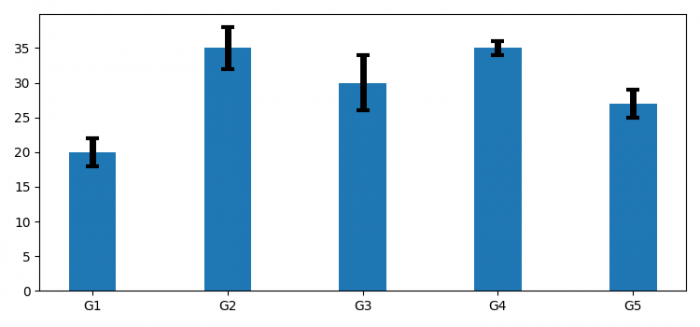# How to increase the thickness of error line in a Matplotlib bar chart?

To increase the thickness of error line in a Matplotlib bar chart, we can use err_kw=dict() with their properties.

## Steps

• Set the figure size and adjust the padding between and around the subplots.
• Make a dictionary of bar details.
• Create a figure and a set of subplots.
• Use bar() method to make a bar plot with yerr and err_kw
• To display the figure, use show() method.

## Example

import matplotlib.pyplot as plt

plt.rcParams["figure.figsize"] = [7.50, 3.50]
plt.rcParams["figure.autolayout"] = True

bar_details = {
"labels": ['G1', 'G2', 'G3', 'G4', 'G5'],
"men_means": [20, 35, 30, 35, 27],
"men_std": [2, 3, 4, 1, 2],
"width": 0.35
}

fig, ax = plt.subplots()

ax.bar(bar_details["labels"], bar_details["men_means"], bar_details["width"],
yerr=bar_details["men_std"], label='Men',
error_kw=dict(lw=5, capsize=5, capthick=3))

plt.show()

## Output